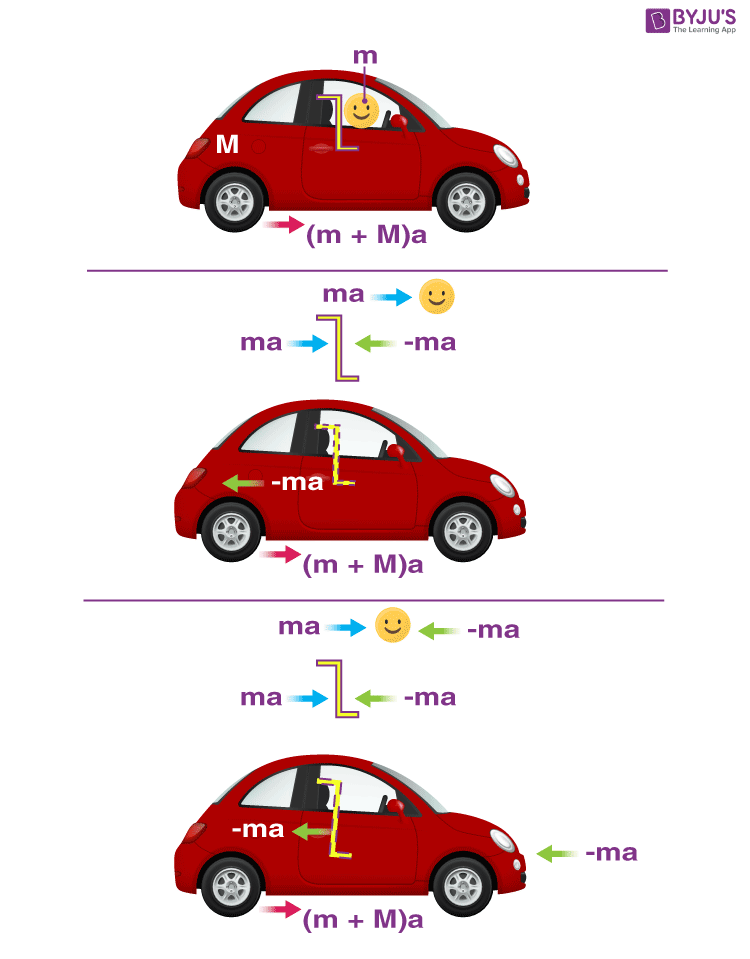# Pseudo Forces- FAQ in JEE Mains

Pseudo forces are also known as fictitious forces come into existence when a body is observed from a non-inertial frame of reference. They are a little difficult to understand and visualize so let’s look at some of the most frequently asked questions related to this topic.## Important Question For Pseudo Forces

1. What is a pseudo force?

Suppose two people A and B are sitting in an accelerated bus and C observes both of them from the ground. So if C draws a free body diagram of B, it will have a net force that gives acceleration. But if A draws a free body diagram for B then for him B is at rest so to counter the force shown by A he needs a fictitious force in his frame. That force is known as pseudo force.

2.  Is centripetal force always equal to centrifugal force?

This is not necessary. If we are in a frame of reference from where the body is at rest as long as the radial direction is concerned the two forces will be equal. But if there is a radial acceleration in a body with respect to a frame then in that frame centripetal force will not be equal to centrifugal force.

3. Is work done by these forces to be considered for the work-energy theorem?

When we are applying the work-energy theorem from a non-inertial frame of reference we need to take into account the work done by pseudo forces.

4. How can these forces help in problem-solving?

Suppose we have a small block that is moving up a wedge that is accelerating with some acceleration. We need to find the time when the small block reaches the top of the wedge. Analysing this situation is much simpler if we are in the wedge frame of reference,  in that case, the Free Body Diagram will have an additional pseudo force as the wedge is accelerated.